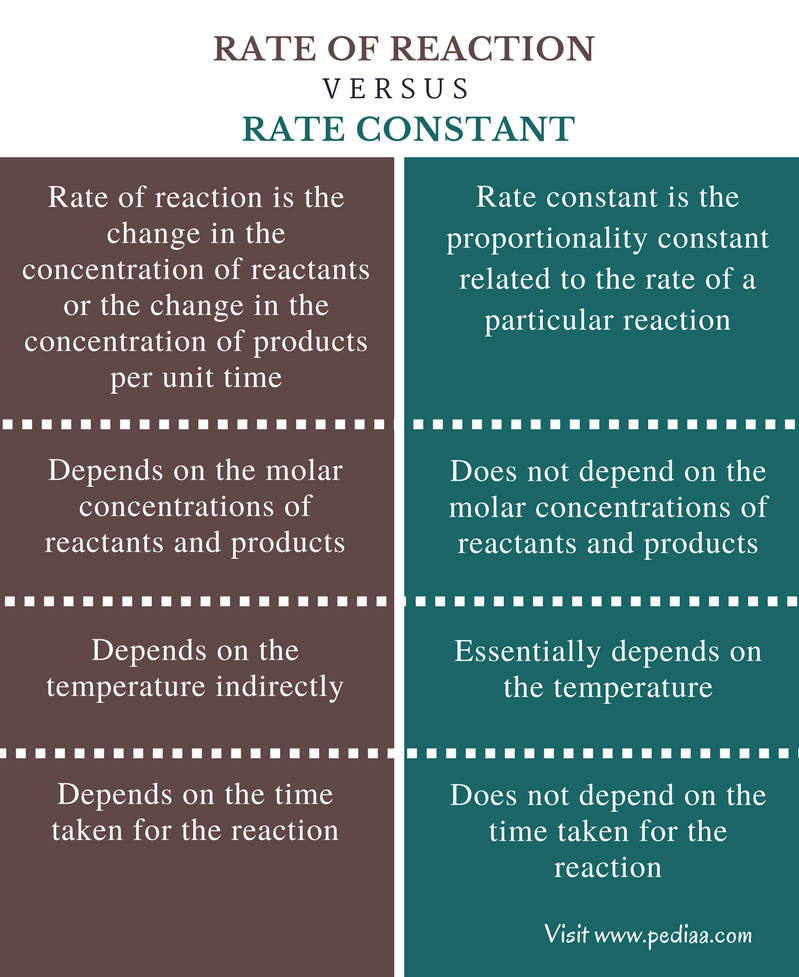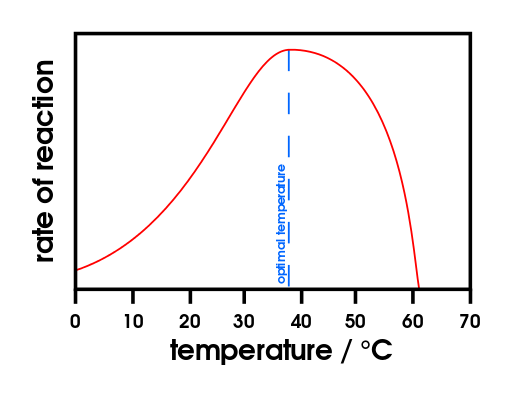# Difference Between Rate of Reaction and Rate Constant

## Main Difference – Rate of Reaction vs Rate Constant

A chemical reaction essentially includes products and reactants. Apart from that, there are some important conditions needed for a chemical reaction to proceed until the completion. Such of these conditions include proper temperature and pressure, ionic strength, etc. However, each and every chemical reaction can be explained using the two terms: the rate of reaction and rate constant. The rate of reaction describes the rate at which the reaction proceeds and rate constant quantifies the rate of a reaction. The main difference between rate of reaction and rate constant is that rate of reaction is the change of the concentration of reactants or the change in concentration of products per unit time whereas rate constant is the proportionality constant related to the rate of a particular reaction.

### Key Areas Covered

1. What is Rate of Reaction

– Definition, Properties, Examples
2. What is Rate Constant

– Definition, Properties, Examples
3. What is the Difference Between Rate of Reaction and Rate Constant

– Comparison of Key Differences

Key Terms: Ionic strength, Products, Rate Constant, Rate of Reaction, Reactants## What is Rate of Reaction

The rate of reaction or the reaction rate is the change in the concentration of reactants or the change in the concentration of products per unit time. This can be obtained by two ways. One is by dividing the concentration of reactants consumed during the reaction from the time elapsed for that consumption. The other method is by dividing the concentration of products formed at the end of the reaction from the time elapsed for that formation. This can be shortened as below.

Rate = [Concentration] / Time

But most of the times, all the reactants are not consumed for the reaction. Therefore, the concentration of the components is taken as the “change of the concentration” at a particular time period. This is given by the symbol Δ. If the concentrations are measured when the time is t1 and then at t2, then the time taken for the reaction is (t2-t1) = time elapsed (Δt). Therefore, the time is taken as Δt. Then, the rate of reaction can be measured even before the completion of the reaction.

Rate =  Δ[reactant] / Δ time = Δ[product] / Δ time

Let us consider a reaction between A and B that gives the product C.

A   +    B    →    C

For the above reaction, the rate of the reaction can be measured by determining the change of concentration of A, B or C.

Rate = – Δ [A] / Δt

Rate = – Δ [B] / Δt

Rate = Δ[C] / Δt

Note that there is a minus mark in front of the concentrations of A and B. That is used to indicate the decreasing of the reactants during the time period of Δt. But there is no minus mark in front of the concentration of C. This is because C is not consumed but is produced so the concentration of C increases throughout the reaction.Figure 1: The graph of rate of reaction vs temperature

The above graph shows the dependence of the rate of reaction on the temperature of an enzymatic reaction. The optimal temperature is the temperature at which the reaction rate is at its peak.

## What is Rate Constant

Rate constant is the proportionality constant related to the rate of a particular reaction. It depends on the temperature of the system. The rate constants give an idea about the rate of a reaction. The symbol for the rate constant is “k”. For example, for the reaction between A and B that gives the product C,

Rate        =      – Δ [A] / Δt

∴     Rate        α     [A]

Rate         =    – Δ [B] / Δt

∴     Rate        α     [B]

The above relationships can be used to build an equation for the rate of the reaction as below.

Rate = k[A]a[B]b

where,

k is the rate constant.

[A] is the concentration of A

[B] is the concentration of B

a is the order of the reaction with respect to A

b is the order of the reaction with respect to B

For a particular temperature, the rate constants have a definite value that will change according to the changes in temperature. This temperature dependence is given by the equation called “Arrhenius equation”.

K = Ae-(EA/RT)

where,

K is the rate constant

A is the pre-exponential factor

EA is the activation energy for the reaction

R is the universal gas constant

T is the temperature of the system

This equation indicates the effect of temperature change on rate constant as well as the effect of a catalyst. Increasing the temperature increases the rate constant. The addition of a catalyst to the reaction mixture decreases the activation energy and increases the rate constant.

## Difference Between Rate of Reaction and Rate Constant

### Definition

Rate of Reaction: Rate of reaction is the change in the concentration of reactants or the change in the concentration of products per unit time.

Rate Constant: Rate constant is the proportionality constant related to the rate of a particular reaction.

### Molar Concentration

Rate of Reaction: The rate of reaction depends on the molar concentrations of reactants and products.

Rate Constant: The rate constant does not depend on the molar concentrations of reactants and products.

### Temperature

Rate of Reaction: Rate of reaction depends on the temperature indirectly.

Rate Constant: Rate constant essentially depends on the temperature.

### Time

Rate of Reaction: The rate of reaction depends on the time taken for the reaction.

Rate Constant: The rate constant does not depend on the time taken for the reaction.

### Conclusion

The rate of reaction and rate constant are very important in determining the best conditions (such as temperature) for a particular chemical reaction. Then it would be easy to handle reactions and can get the optimum amounts of product in a short time period.  Therefore, it is very important to understand the properties and the differences between rate of reaction and rate constant.

##### References:

1.”Rate constants and the arrhenius equation.” Rate constants and the arrhenius equation. N.p., Oct. 2002. Web. Available here. 14 July 2017.
2.”Reaction rate.” Encyclopædia Britannica. Encyclopædia Britannica, inc., n.d. Web. Available here. 14 July 2017.

##### Image Courtesy:

1.”Effect of temperature on enzymes” By domdomegg – Own work (CC BY 4.0) via Commons Wikimedia